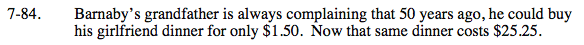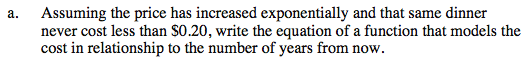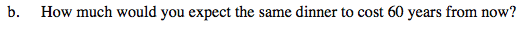### Home > INT3 > Chapter Ch7 > Lesson 7.2.3 > Problem7-84

7-84.
1. Barnaby’s grandfather is always complaining that 50 years ago, he could buy his girlfriend dinner for only $1.50. Now that same dinner costs$25.25. Homework Help ✎

1. Assuming the price has increased exponentially and that same dinner never cost less than $0.20, write the equation of a function that models the cost in relationship to the number of years from now. 2. How much would you expect the same dinner to cost 60 years from now?Begin by defining variables. Let x = the number of years Let y = the cost of a dinner You need to write an equation of the form y = abx + k. Use the points (−50, 1.50) and (0, 25.25). The horizontal asymptote (or vertical shift in this case) is y = 0.20 (or k = 0.20).Let x = 60 in your equation from part (a). Answers vary depending on precision used, about$874.64.